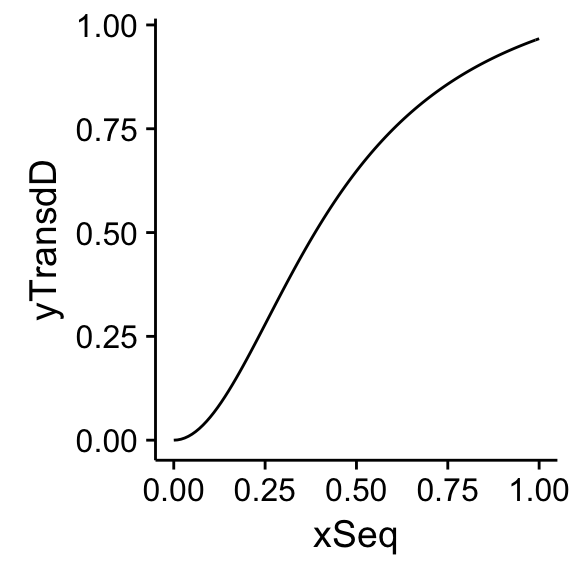Let’s suppose that in each trial we present one stimulus to the left or right of the fixation point and the observer needs to decide in which location the stimulus was presented. We use five contrast levels and for each level we present the stimulus 100 times. Here some hypothetical data

library('ggplot2')
n<-100
x<-c(0.05,0.10,0.15,0.20,0.25) # contrast
k<-c(59,56,69,90,96) # number of times that the observer reports that can see the stimulus
y<-k/n
dat<-data.frame(x,k,y)

p<-ggplot()+
geom_point(data=dat,aes(x=x,y=y))
p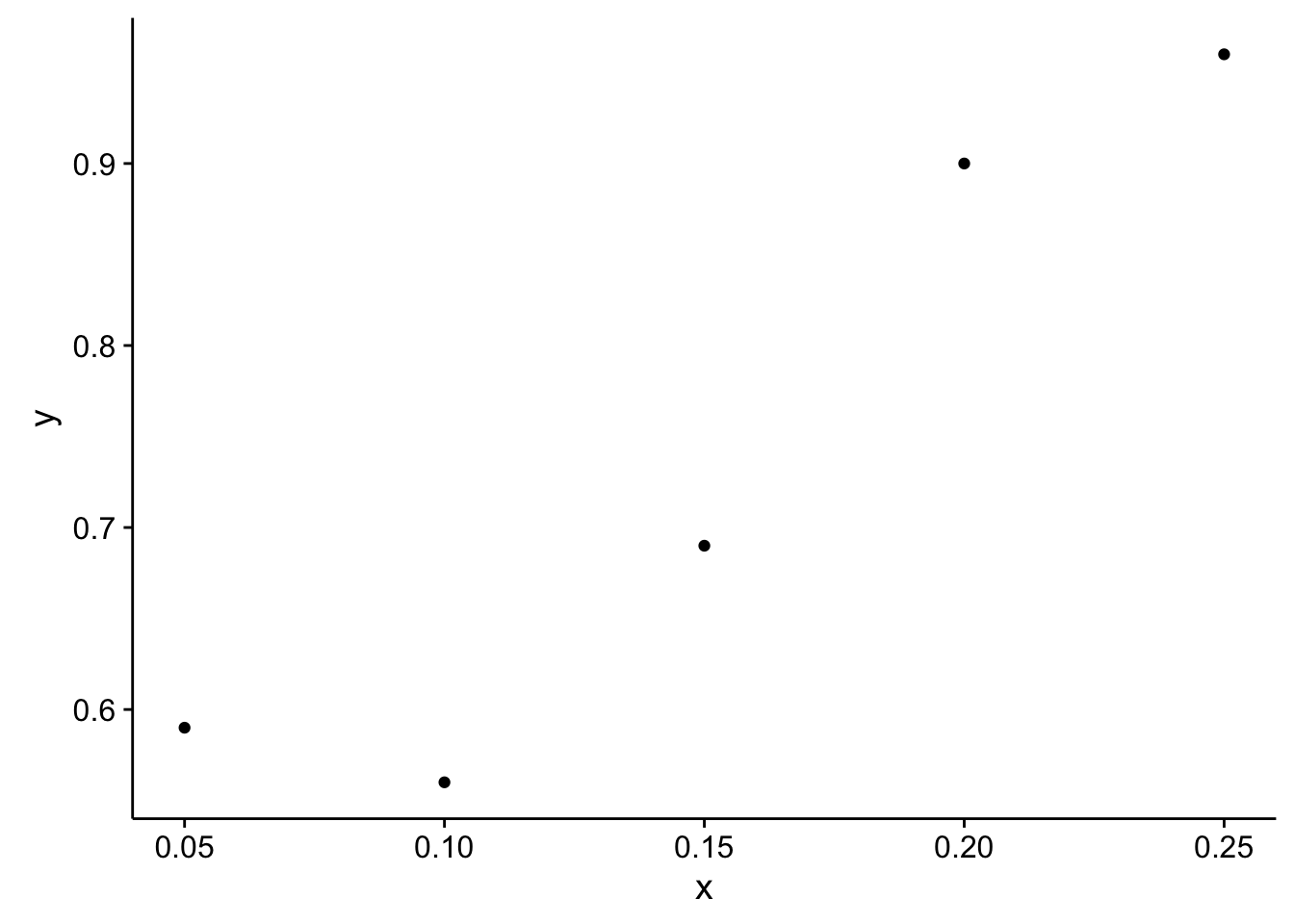Instead of chosing a shape for the psychometric function, we choose a shape for the transduction of contrast to perceived contrast. From the transducer function and assuming constant noise (additive), we will recover the shape of the psychometric function.

First, let’s choose a general expression for the transduction of contrast $$\mu$$ $\mu(x)=\frac{p_1 x^{p_3}}{p_2+x^{p_3}}$

mu<-function(x,p){
f<-function(x) {   #naka-rushton shape
num<-p*x^p; den<-x^p+p
return(num/den)
}
sapply(x,function(x) f(x))
}

Let’s plot $$\mu$$ for some arbitrary parameters as examples

xSeq<-seq(0,1,.01)
yTransdEx1<-mu(xSeq,c(1,1,1))
example1<-data.frame(xSeq,yTransdEx1)
qplot(xSeq,yTransdEx1,example1,geom='line')
## Warning: Ignoring unknown parameters: NA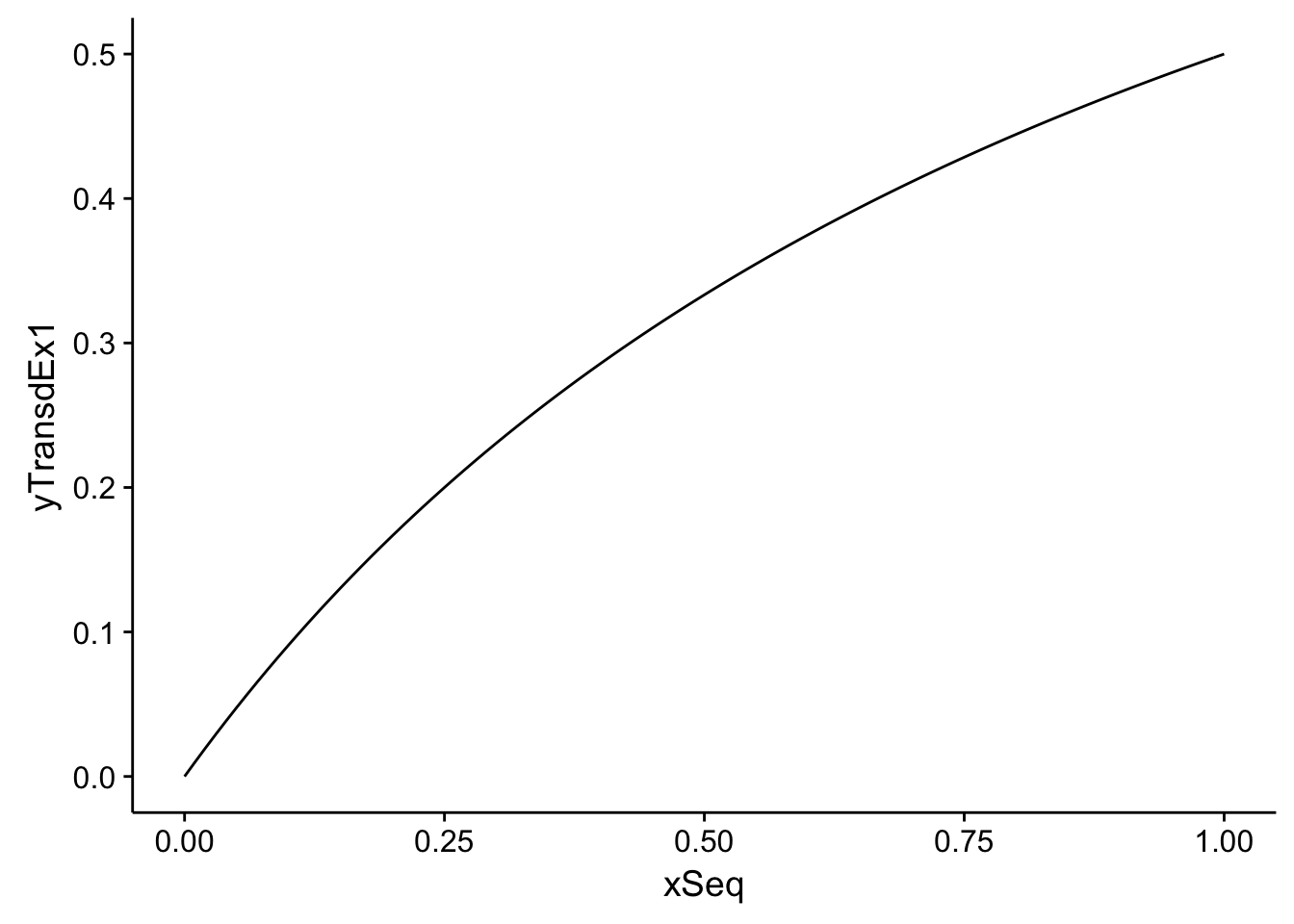yTransdEx2<-mu(xSeq,c(1,1,2))
example2<-data.frame(xSeq,yTransdEx2)
qplot(xSeq,yTransdEx2,example2,geom='line')
## Warning: Ignoring unknown parameters: NA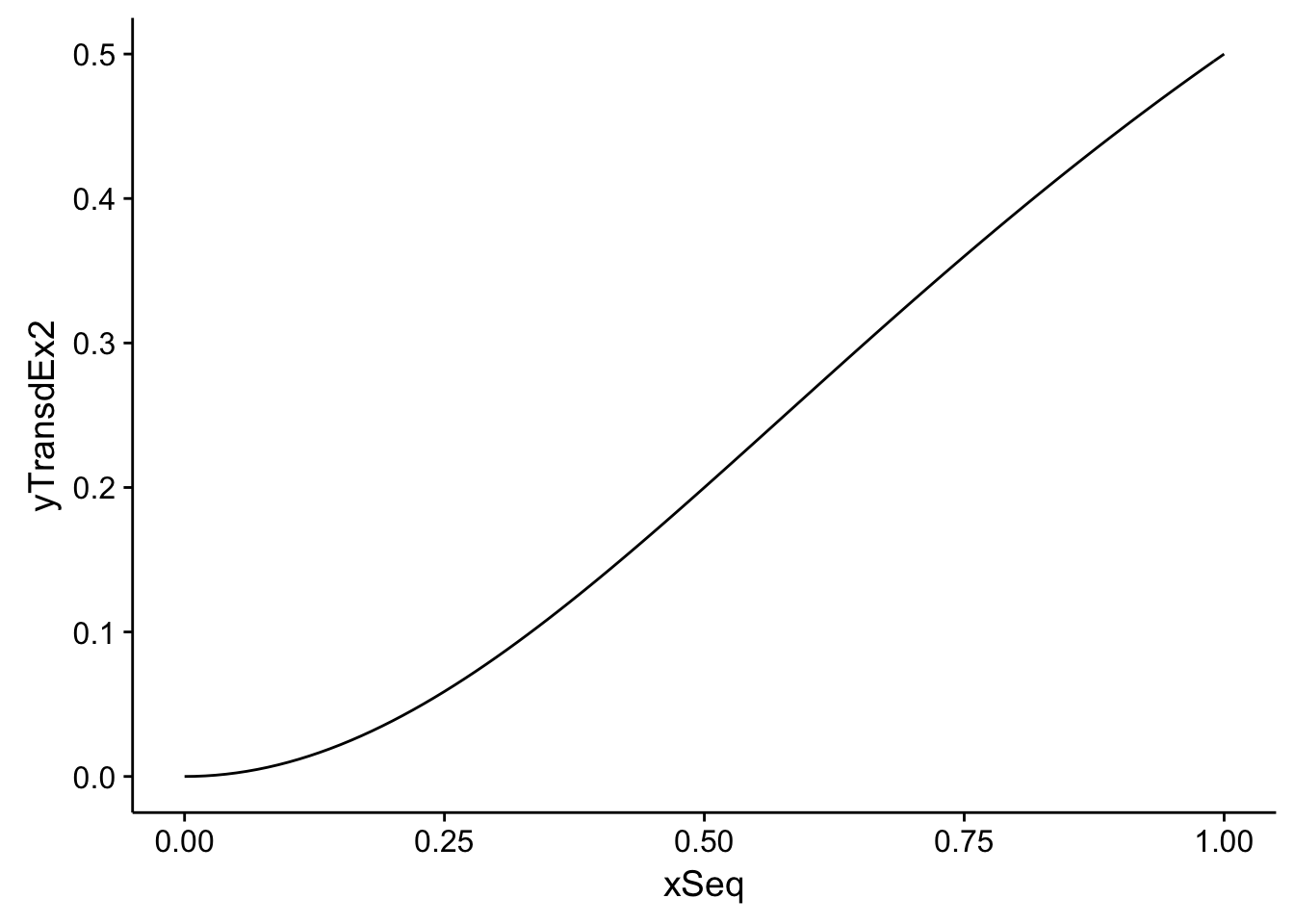We assume that the perceived contrast elicited by the stimulus containing zero contrast is described by a random variable $$R_p$$ distributed normally with mean $$\mu(0)$$ and variance $$\sigma^2$$ and that the perceived contrast response elicited by the stimulus containing non-zero contrast is described by a random variable $$R_v$$ distributed normally with mean $$\mu(x)$$ and the same variance $$\sigma^2$$.

We construct the random variable $$D=R_v-R_p$$, which is distributed normally with mean $$\mu(x)-\mu(0)$$ and variance $$2\sigma^2$$. The observer will choose the non-zero contrast stimulus when $$D>0$$, which is $$1-F(0)$$ where $$F$$ is the cumulative distribution function. That is,

$P(D>0)=1 - \int_{-\infty}^{0}N(D;\mu(x)-\mu(0),2\sigma^2) dD$

where N is the normal distribution. Given that the D is just a function of the contrast $$x$$, the above expression is just an expression of the psychometric function.

psychoFromTransd0<-function(x,p) {
# let's allow p to contain the parameters of the transducer and the noise
pNoise<-tail(p,1)
sapply(mu(x,pTransd)-mu(0,pTransd),
function(z) 1 - pnorm(0,mean=z,sd=sqrt(2)*pNoise))
}

Let’s plot two psychometric functions for some arbitrary parameters as examples

ySeqEx1<-psychoFromTransd0(xSeq,c(1,1,1,.1))
examplePsycho1<-data.frame(xSeq,yTransdEx1)
qplot(xSeq,ySeqEx1,examplePsycho1,geom='line')
## Warning: Ignoring unknown parameters: NA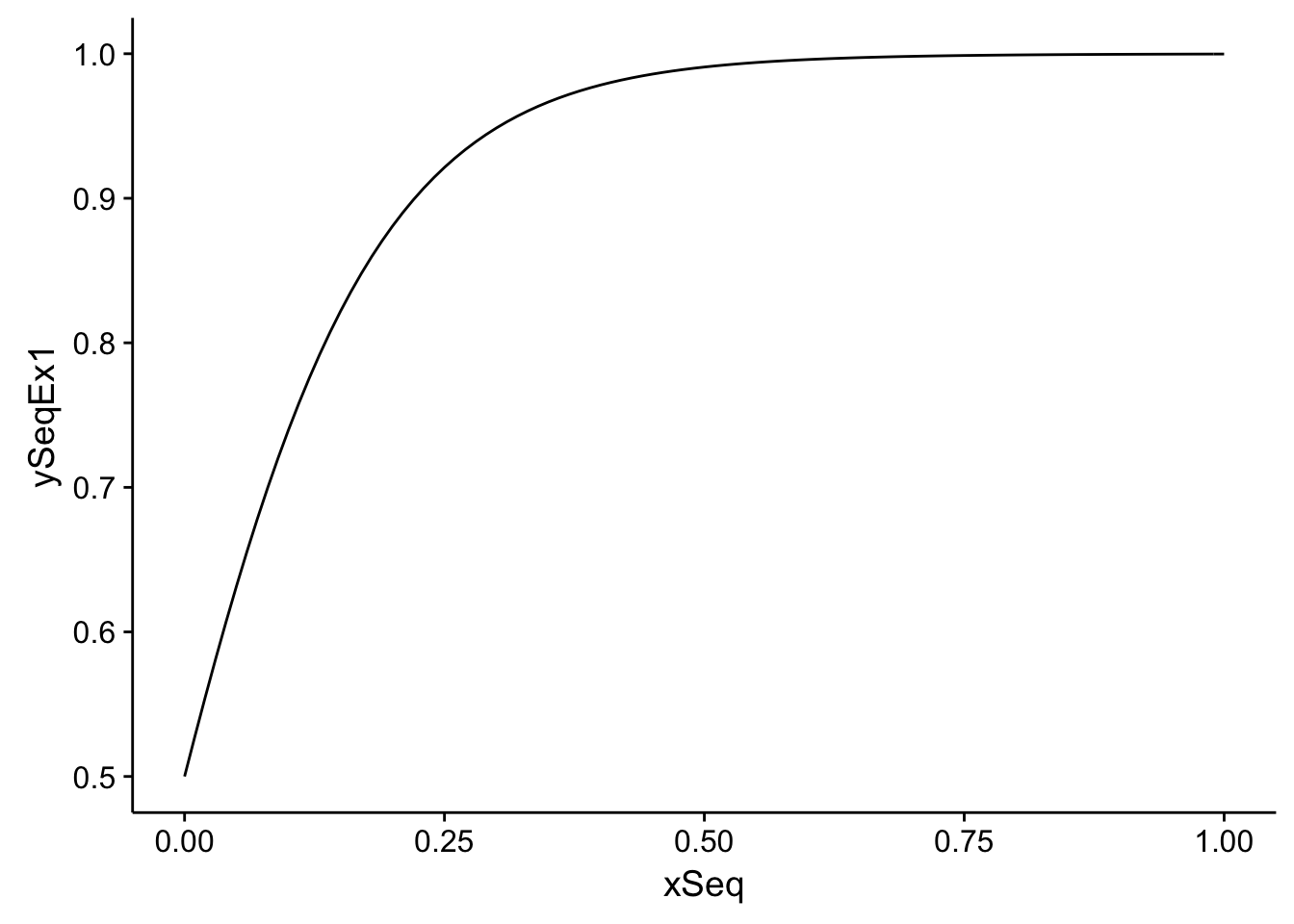ySeqEx2<-psychoFromTransd0(xSeq,c(1,1,2,.3))
examplePsycho2<-data.frame(xSeq,yTransdEx2)
qplot(xSeq,ySeqEx2,examplePsycho2,geom='line')
## Warning: Ignoring unknown parameters: NA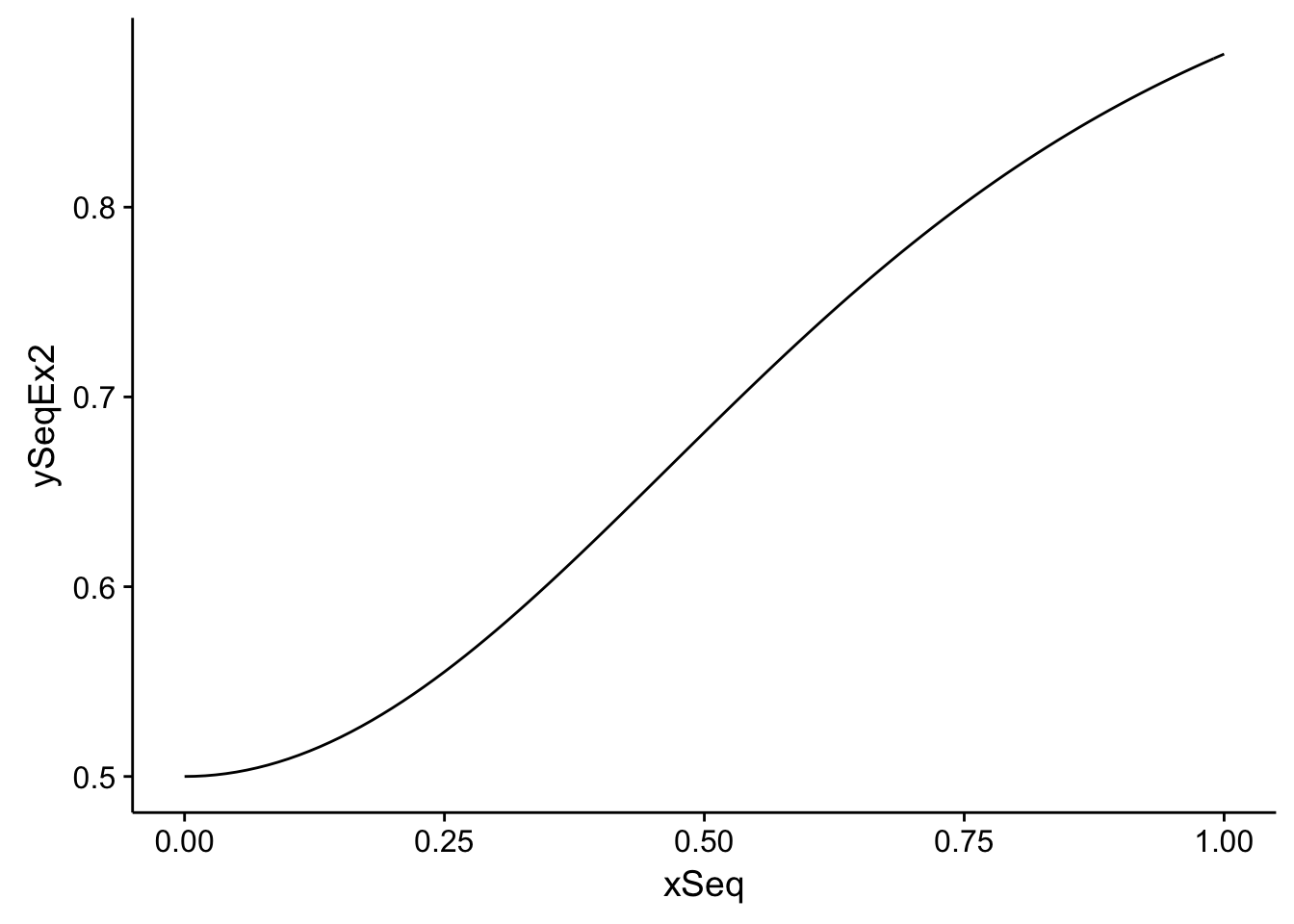Let’s find the best parameters using maximum likelihood estimation

negLogL<-function(p,d,fun) {
phi<-fun(d$x,p) -sum( d$k*log(phi)+(n-d$k)*log(1-phi) ) } MLEparameters<-optim(c(1,1,1,.1), negLogL,d=dat,fun=psychoFromTransd0)$par
MLEparameters
##  2.13567206 0.05649665 2.74941451 0.23028307

and plot the psychometric function

ySeq<-psychoFromTransd0(xSeq,MLEparameters)
curve<-data.frame(xSeq,ySeq)
p<-p+geom_line(data=curve,aes(x=xSeq,ySeq))
p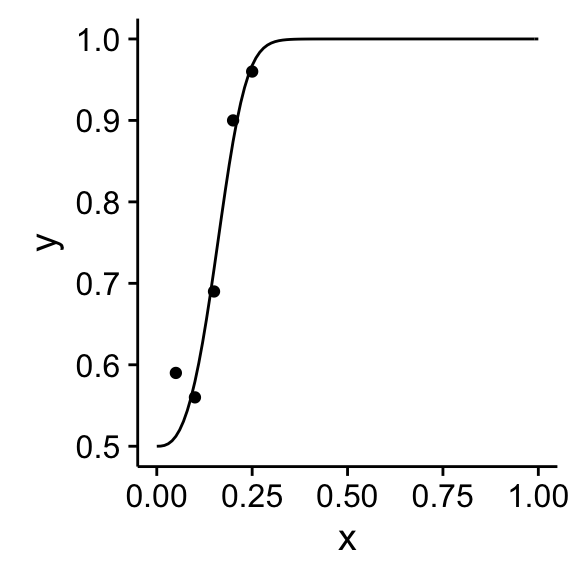and the estimated transducer

yTransd<-mu(xSeq,MLEparameters)
qplot(xSeq,yTransd,data.frame(xSeq,yTransd),geom='line')
## Warning: Ignoring unknown parameters: NA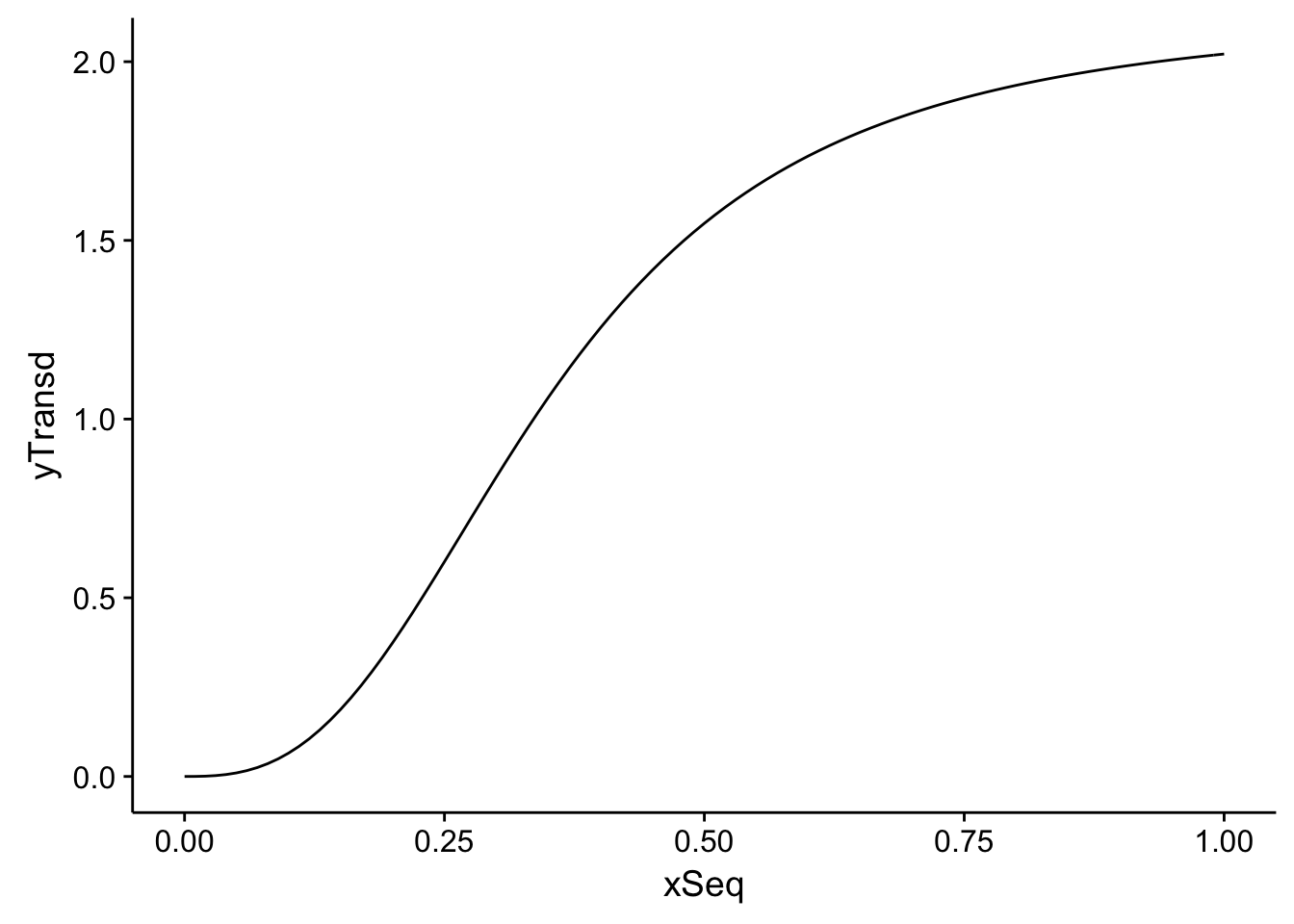We might have used a simpler transduction of contrast, such as a linear transduction. In this case, it could be easily demonstrated that the shape of the psychometric function is a cumulative normal (Kingdom and Prins, 2009).

## Fitting several psychometric functions for discrimination

Typically, we fit psychometric functions that have only two parameters. Here, instead we used four (the three parameters of the transducer and the noise parameter). Maybe too many parameters to fit a psychometric function. So, this approach to fit psychometric functions, maybe makes more sense when we don’t just measure detection, but discrimination of contrast for several contrasts (pedestals).

Let’s suppose that in each trial we present one stimulus to the left and one to the right of the fixation point and the observer needs to decide which stimulus have higher contrast. We fix the contrast of one of them (pedestal) and change the contrast of the other one (variable) in each trial. For the variable stimulus, we use five contrast levels and for each level we present the stimulus 100 times. Here some hypothetical data for 4 pedestal contrast

library('ggplot2')
n<-100
pedestal<-c(0,.25,.5,.75)
x1<-pedestal+c(0.05,0.10,0.15,0.20,0.25) # contrast of the variable
k1<-c(59,62,70,82,90) # number of times that the observer reports that the variable has higher contrast
y1<-k1/n
dat1<-data.frame(x=x1,k=k1,y=y1,pedestal=pedestal)

x2<-pedestal+c(0.05,0.10,0.15,0.20,0.25)
k2<-c(58,80,90,95,97)
y2<-k2/n
dat2<-data.frame(x=x2,k=k2,y=y2,pedestal=pedestal)

x3<-pedestal+c(0.05,0.10,0.15,0.20,0.25)
k3<-c(50,62,70,79,86)
y3<-k3/n
dat3<-data.frame(x=x3,k=k3,y=y3,pedestal=pedestal)

x4<-pedestal+c(0.05,0.10,0.15,0.20,0.25)
k4<-c(50,62,65,67,75)
y4<-k4/n
dat4<-data.frame(x=x4,k=k4,y=y4,pedestal=pedestal)

datD<-rbind(dat1,dat2,dat3,dat4)

pD<-ggplot()+
geom_point(data=datD,aes(x=x,y=y,color=factor(pedestal)))
pD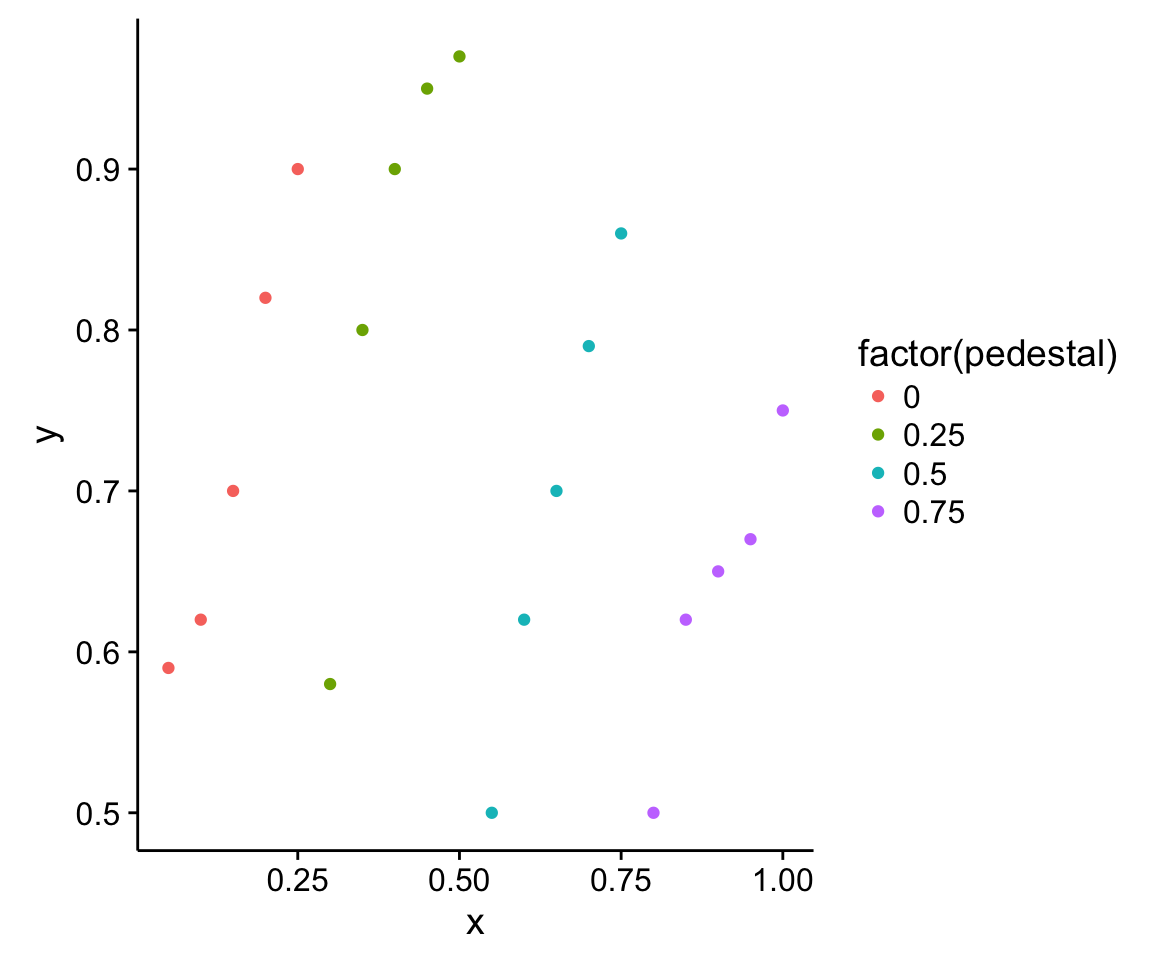We rewrite the function that builds the psychometric function from the transducer to replace the 0 contrast for a general pedestal

psychoFromTransd<-function(x,pedestal,p) {
pNoise<-tail(p,1)
sapply(mu(x,pTransd)-mu(pedestal,pTransd),
function(z) 1- pnorm(0,mean=z,sd=sqrt(2)*pNoise))
}

and change the likelihood function to sum the log likelihood for each pedestal.

library('plyr')
negLogLD<-function(p,d,fun) {
negLogForEachPedestal<-ddply(d,.(pedestal),function(d2){
phi<-fun(d2$x,unique(d2$pedestal),p)
negLog<- -sum( d2$k*log(phi)+(n-d2$k)*log(1-phi))
data.frame(negLog)
})
sum(negLogForEachPedestal$negLog) } MLEparametersD<-optim(c(1,1,1,.1), negLogLD,d=datD,fun=psychoFromTransd)$par
MLEparametersD
##  1.1557587 0.1952639 2.0045113 0.1479516

These are the psychometric functions (we needed just 4 parameters to fit 4 psychometric functions)

curves<-ddply(data.frame(pedestal),.(pedestal),function(d){
xSeq<-seq(d$pedestal,d$pedestal+.25,by=.01)
ySeq<-psychoFromTransd(xSeq,d\$pedestal,MLEparametersD)
data.frame(xSeq,ySeq)
})
pD<-pD+geom_line(data=curves,aes(x=xSeq,ySeq,color=factor(pedestal)))
pD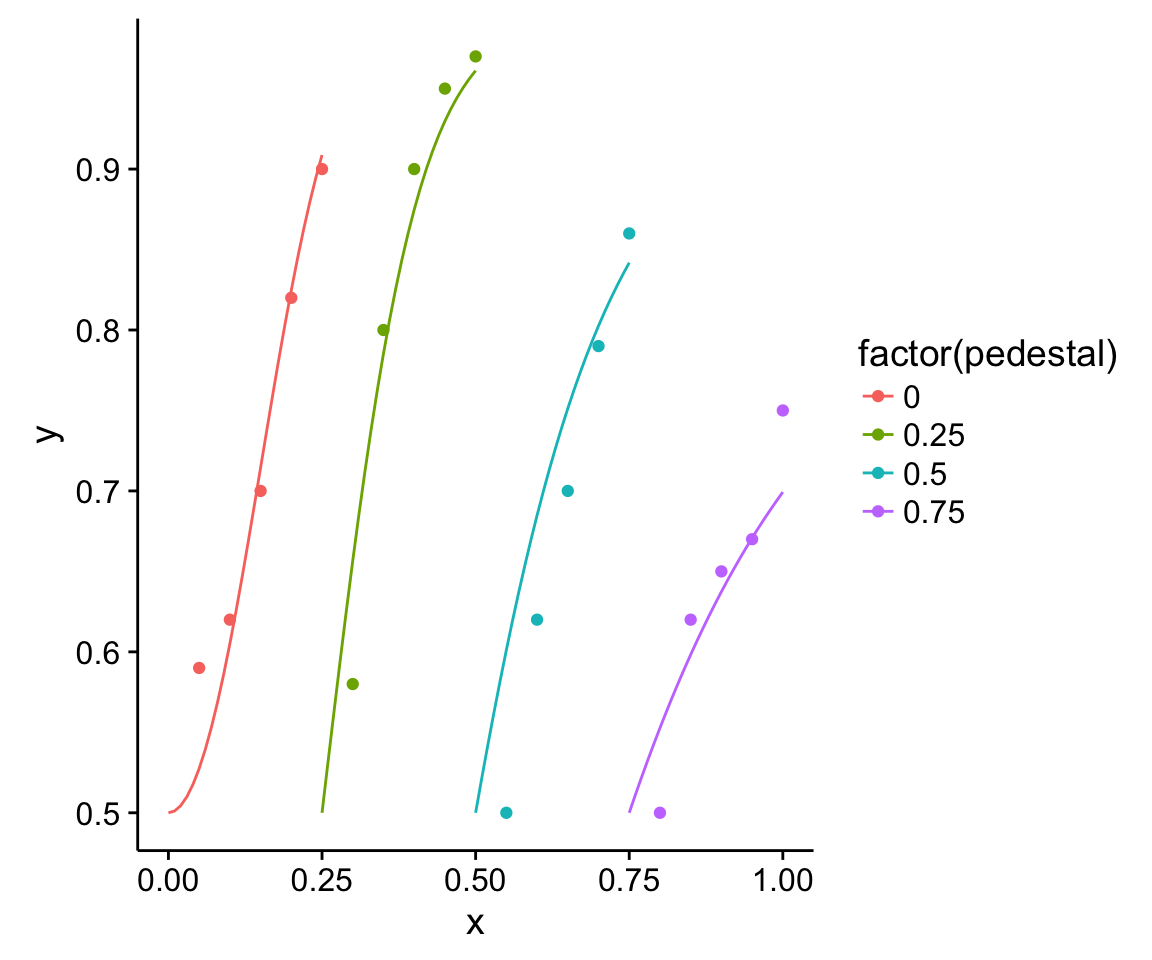and the estimated transducer.

yTransdD<-mu(xSeq,MLEparametersD)
qplot(xSeq,yTransdD,data.frame(xSeq,yTransdD),geom='line')
## Warning: Ignoring unknown parameters: NA